# Complete Graphs

Relevant articles: , , , , , , , , , , , , , , , , , , , , , , .

[hide]

# Complete Graphs

In this section the complete graph on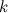$k$ vertices, denoted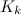$K_k$, is considered. A$K_k$-design of order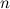$n$ is better known as a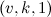$(v,k,1)$-BIBD with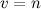$v=n$.

## Spectrum Results

For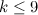$k\leq 9$, Table 1 summarises the known results on the spectrum problem for$K_k$. An explanation of the sources of these results is given in .

Table 1$k$ Spectrum for$K_k$ Possible exceptions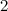$2$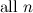${\rm \ all \ }n$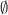$\emptyset$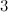$3$$n \equiv 1 {\rm \ or \ } 3 \,({\rm mod \ }6)$$\emptyset$$4$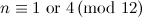$n \equiv 1 {\rm \ or \ } 4 \,({\rm mod \ }12)$$\emptyset$$5$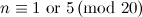$n \equiv 1 {\rm \ or \ } 5 \,({\rm mod \ }20)$$\emptyset$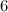$6$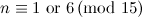$n \equiv 1 {\rm \ or \ } 6 \,({\rm mod \ }15)$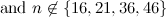${\rm and \ }n \not\in\{16,21,36,46\}$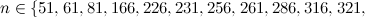$n\in\{51,61,81,166,226,231,256,261,286,316,321,$$346,351,376,406,411,436,441,471,501,561,591,616,$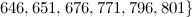$646,651,676,771,796,801\}$$7$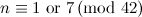$n \equiv 1 {\rm \ or \ } 7 \,({\rm mod \ }42)$${\rm and \ }n \neq 43$$n=42t+1{\rm \ for \ }t\in\{2,3,5,6,12,14,17,19,22,27,33,$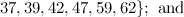$37,39,42,47,59,62\};{\rm \ and}$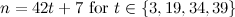$n=42t+7{\rm \ for \ }t\in\{3,19,34,39\}$$8$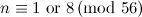$n \equiv 1 {\rm \ or \ } 8 \,({\rm mod \ }56)$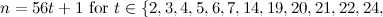$n=56t+1{\rm \ for \ }t\in\{2,3,4,5,6,7,14,19,20,21,22,24,$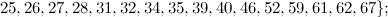$25,26,27,28,31,32,34,35,39,40,46,52,59,61,62,67\};$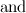${\rm and}$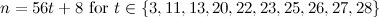$n=56t+8{\rm \ for \ }t\in\{3,11,13,20,22,23,25,26,27,28\}$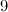$9$$n \equiv 1 {\rm \ or \ } 9 \,({\rm mod \ }72)$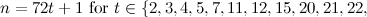$n=72t+1{\rm \ for \ }t\in\{2,3,4,5,7,11,12,15,20,21,22,$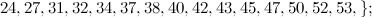$24,27,31,32,34,37,38,40,42,43,45,47,50,52,53,\};$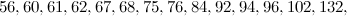$56,60,61,62,67,68,75,76,84,92,94,96,102,132,$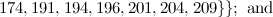$174,191,194,196,201,204,209\}\};{\rm \ and}$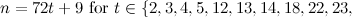$n=72t+9{\rm \ for \ }t\in\{2,3,4,5,12,13,14,18,22,23,$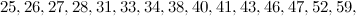$25,26,27,28,31,33,34,38,40,41,43,46,47,52,59,$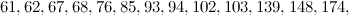$61,62,67,68,76,85,93,94,102,103,139,148,174,$$183,192,202,203,209,229\}$

For$k\geq 10$, the known$K_k$-designs are given by Theorem 1 (and Wilson's Theorem).

Theorem 1

Let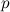$p$ be a prime power and let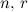$n,\, r$ and$s$ be integers such that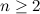$n \geq 2$ and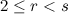$2 \leq r < s$.

• There exists a$K_q$-design of order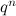$q^n$ (affine geometries) (see ).
• There exists a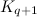$K_{q+1}$-design of order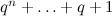$q^n + \ldots + q + 1$ (projective geometries) (see ).
• There exists a$K_{q+1}$-design of order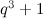$q^3+1$ (unital designs) (see ).
• There exists a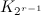$K_{2^{r-1}}$-design of order$2^{r-1}(2^r-1)$ (oval designs) .
• There exists a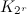$K_{2^r}$-design of order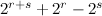$2^{r+s}+2^r-2^s$ (Denniston designs) .

Obvious necessary conditions for the existence of a$K_k$-design of order$n$ are

•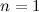$n = 1$ or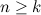$n \geq k$;
•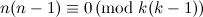$n(n-1)\equiv 0 \,({\rm mod \ }k(k-1))$; and
•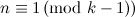$n \equiv 1 \,({\rm mod \ }k-1))$.

Further necessary conditions for the existence of a$K_k$-design of order$n$ are described in the following three theorems.

Theorem 2 

There is no$K_k$-design of order$n$ for any$n$ in the range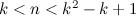$k < n < k^2-k+1$.

Theorem 3 , 

If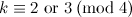$k \equiv 2 {\rm \ or \ } 3 \,({\rm mod \ }4)$ and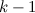$k-1$ is not the sum of two integer squares then there is no$K_k$-design of order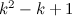$k^2-k+1$ and no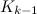$K_{k-1}$-design of order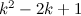$k^2-2k+1$.

Theorem 4 

There is no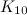$K_{10}$-design of order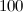$100$ and no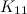$K_{11}$-design of order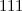$111$.

## Notes

• A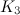$K_3$-design is a Steiner triple system.

## References

1. Abel, R. J. R. Some new BIBDs with λ=1 and 6\leq k\leq 10, J. Combin. Des. 4, 27–50 (1996).
2. Abel, R. J. R., Bluskov, I., Greig, M., and de Heer, J. Pair covering and other designs with block size 6, J. Combin. Des. 15, 511 (2007).
3. Abel, R. J. R. and Greig, M. BIBDs with small block size, CRC Press Series on Discrete Mathematics and its Applications, The CRC handbook of combinatorial designs, ed. 1, CRC Press, Boca Raton, FL, 41–47 (1996).
4. Abel, R. J. R. and Greig, M. Some new RBIBDs with block size 5 and PBDs with block sizes \equiv 1\bmod 5, Australas. J. Combin. 15, 177–202 (1997).
5. Abel, R. J. R. and Greig, M. BIBDs with small block size, CRC Press Series on Discrete Mathematics and its Applications, The CRC handbook of combinatorial designs, ed. 2, CRC Press, Boca Raton, FL, 72–79 (2007).
6. Abel, R. J. R. Some new BIBDs with block size 7, J. Combin. Des. 8, 146–150 (2000).
7. 7.0 7.1 Adams, P., Bryant, D., and Buchanan, M. A survey on the existence of G-designs, J. Combin. Des. 16, 373–410 (2008).
8. 8.0 8.1 Assmus Jr., E. F. and Key, J. D. Designs and their codes, Cambridge Tracts in Mathematics, Vol. 103, Cambridge:Cambridge University Press, (1992).
9. 9.0 9.1 Bose, R. C. and Shrikhande, S. S. On the construction of sets of mutually orthogonal Latin squares and the falsity of a conjecture of Euler, Trans. Amer. Math. Soc. 95, 191–209 (1960).
10. 10.0 10.1 Bruck, R. H. and Ryser, H. J. The nonexistence of certain finite projective planes, Canadian J. Math. 1, 88–93 (1949).
11. Buratti, M. and Zuanni, F. \$G-invariantly resolvable Steiner 2-designs which are 1-rotational over G, Bull. Belg. Math. Soc. Simon Stevin, 5, 221–235 (1998).
12. 12.0 12.1 Chowla, S. and Ryser, H. J. Combinatorial problems, Canadian J. Math. 2, 93–99 (1950).
13. 13.0 13.1 Denniston, R. H. F. Some maximal arcs in finite projective planes, J. Combinatorial Theory, 6, 317–319 (1969).
14. 14.0 14.1 Fisher, R. A. An examination of the different possible solutions of a problem in incomplete blocks, Ann. Eugenics, 10, 52–75 (1940).
15. Greig, M. Recursive constructions of balanced incomplete block designs with block size of 7, 8 or 9, Ars Combin. 60, 3–54 (2001).
16. Hanani, H. The existence and construction of balanced incomplete block designs, Ann. Math. Statist. 32, 361–386 (1961).
17. Houghten, S. K., Thiel, L. H., Janssen, J., and Lam, C. W. H. There is no (46,6,1) block design, J. Combin. Des. 9, 60–71 (2001).
18. Janko, Z. and Tonchev, V. D. New designs with block size 7, J. Combin. Theory Ser. A, 83, 152–157 (1998).
19. Kirkman, T. P. On a problem in combinations, Cambridge and Dublin Math. J. 2, 191–204 (1847).
20. 20.0 20.1 Lam, C. W. H., Thiel, L., and Swiercz, S. The nonexistence of finite projective planes of order 10, Canad. J. Math. 41, 1117–1123 (1989).
21. Mills, W. H. BIBDs with k=6 and λ=1, Math. Appl. Computational and constructive design theory, Kluwer Acad. Publ. Dordrecht, 189–226 (1996).
22. 22.0 22.1 22.2 Wallis, W. D. Combinatorial designs, Monographs and Textbooks in Pure and Applied Mathematics, Vol. 118, New York:Marcel Dekker Inc. (1988).
23. Wilson, R. M. Decompositions of complete graphs into subgraphs isomorphic to a given graph, Proceedings of the Fifth British Combinatorial Conference (Univ. Aberdeen, Aberdeen, 1975), Congressus Numerantium, No. XV, Utilitas Math., Winnipeg, Man. 647–659 (1976).